/

### Extreme Value Theory 3. Main Block maxima results and the Fisher-Tippett, Gnedenko theorem

Next page

As noted in the Introduction, ‘block maxima’ results are the more traditional variant of EVT but are also less useful for risk management purposes as they are less directly relevant to the task of, say, estimating VaR’s at extreme threshold levels. However, it is conventional to discuss these first, so we also develop EVT in this manner.

Suppose we are interested in statistics applicable to a set of portfolio losses measured over time. We assume that these losses are random variables. These losses will be a series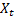, say. We will first assume that the losses are independent and identically distributed (‘i.i.d’) but later we will relax this assumption. We will also assume that the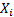are continuous random variables.

The role of the generalised extreme value (GEV) distribution in the theory of extremes

Is analogous to the role the normal distribution plays within the Central Limit Theorem (CLT). With the CLT we have to normalise the data for a limiting distribution to appear. Specifically, if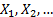are iid with a finite variance and if we write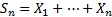then the CLT indicates that appropriately normalised sums,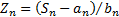converge in distribution to the standard normal, i.e. the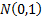, distribution astends to infinity. By ‘normalise’ we here mean a sequence of normalising constants not dependent on any particularbut dependent merely onand on the parameters characterising the distribution from which they are all drawn. For the CLT the normalising constants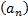and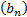are defined by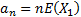and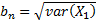. In mathematical notation we have: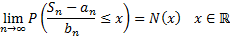where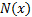is the cumulative distribution function of the unit normal distribution.

Block maxima results focus on suitably normalised maxima of discrete sets of. So, suppose each block consists ofelements (so the’th block involves elements numbered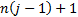to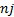(if the first entry in the series is numbered entry 1). We calculate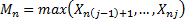and we are interested in the distributional form of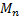(appropriately normalised) as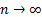. If the available observed data involves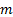such blocks, i.e. is of length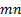, say, then we will have onlydifferent (independent) values of. In some loose sense only ‘one’ data point from each block drivesand any information implicit in the remainder is thrown away by focusing merely on these maxima (although of course all in some underlying sense influence). Thus the approach appears likely to make relatively inefficient use of the available data when applied to real life data series, ifis large.

Suppose the cumulative distribution function of eachis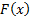, then because they are i.i.d. we will have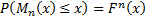The main block maxima EVT result is then as follows:

(1)          Suppose that there are real sequences of numbers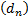and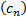, where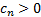for allsuch that: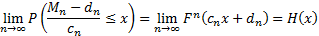for some non-degenerate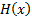(by non-degenerate we mean that the limiting distribution is not concentrated onto a single point).

(2)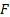is then said to be in the maximum domain of attraction of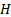, written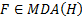.

(3)          The Fisher-Tippett, Gnedenko Theorem states that iffor some non-degenerate distribution functionthen(when appropriately standardised) must represent a generalised extreme value (GEV) distribution,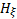, for some value of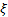. Such a distribution has a distribution function: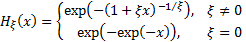where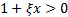.

A (non-standardised) three-parameter family is obtained by defining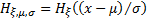for a location parameter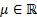and a scale parameter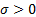. It is always possible to chooseandso that the resulting distribution takes the standard form. Some commentators replaceby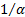to make the link with Pareto distributions clearer (see threshold exceedance results). If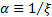is positive then it is known as the tail index, for reasons set out below.

The GEV is ‘generalised’ in the sense that it subsumes three types of distribution which are known by other names, i.e.:

 Value of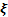Distributional type Distributional form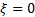Gumbel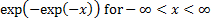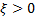Fréchet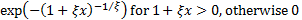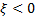Weibull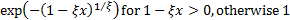The Weibull distribution is a short-tailed distribution with a so-called finite right endpoint,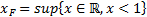. The Gumbel and Fréchet distributions have infinite right end points, but the decay in the tail of the Fréchet distribution is much slower than for the Gumbel distribution.